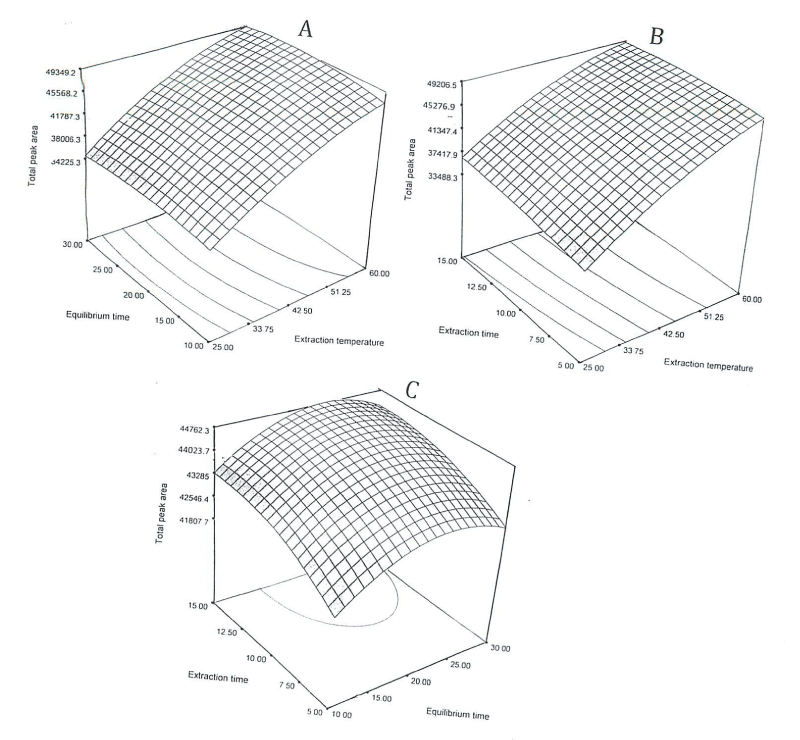Figure 1: Response surface plot for: (A) total area versus equilibrium time (min) and extraction temperature (°C), with a constant extraction time equal to 10 min; (8) total area versus extraction time (min) and extraction temperature (°C), with a constant equilibrium time equal to 20 min; (C) total area versus extraction time (min) and equilibrium time (min), with a constant extraction temperature equal to 42.50°C